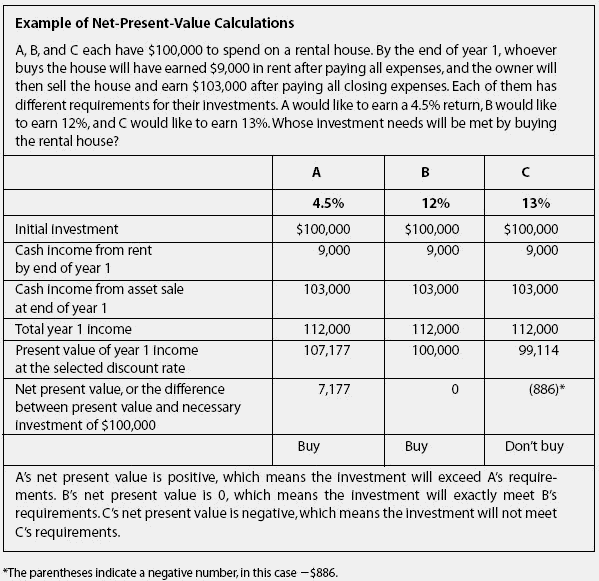# net present value

(redirected from Present Value, Net)
Also found in: Dictionary.

## Net present value (NPV)

The present value of the expected future cash flows minus the cost.

## Net Present Value

A measure of discounted cash inflow to present cash outflow to determine whether a prospective investment will be profitable. For example, if a dentist wishes to purchase a new dental practice, he may calculate the net present value over a number of years to see if he will recover his investment in a reasonable period of time. If the ask price for the dental practice is \$500,000, this is the present cash outflow used in the calculation. If the discounted cash inflow over, say, two years, is greater than or equal to \$500,000, then the investment will likely be profitable. See also: Present value.

## net present value

The discounted value of an investment's cash inflows minus the discounted value of its cash outflows. To be adequately profitable, an investment should have a net present value greater than zero. For investment in securities, the initial cost is usually the only outflow.

## net present value

see INVESTMENT APPRAISAL, DISCOUNTED CASH FLOW.
Collins Dictionary of Business, 3rd ed. © 2002, 2005 C Pass, B Lowes, A Pendleton, L Chadwick, D O’Reilly and M Afferson

## net present value

see DISCOUNTED CASH FLOW.
Collins Dictionary of Economics, 4th ed. © C. Pass, B. Lowes, L. Davies 2005

## net present valueAn analytical tool for evaluating whether or not to purchase an investment. The tool does not tell you if an investment is good or bad;it tells you if the investment will meet your predetermined objectives or not.

• Critical to defining your objectives is setting the equivalent of an interest rate you would like to earn on your initial cash investment. This is called the discount rate. The discount rate may change from investment to investment, depending on your assessment of the risk. The safest investment is an FDIC-insured savings account, but it returns the lowest interest rate. You, the investor, decide what rate you would like to earn. You use the net-present-value tool to calculate whether a particular investment will earn the rate you want.

• Having said all that, the official definition of net present value is as follows: using a preselected discount rate, net present value is the present value of all cash incomes, less the present value of all cash outflows (including initial investment). If this is not clear, it will become so with the example below.

• If the net present value is 0 or a positive number, the investor should go forward. If the answer is negative for the discount rate selected, then the investment should not be made because it will not meet the investor's objectives, not because it is a “bad investment” in the ordinary sense of that phrase.

• The Excel formula for present value is pv (rate, cashflow, cashflow, cashflow)
‘'Rate” is the cell with the interest rate the investor would like to earn. Each of the “cashflow” entries is a cell address for cash flows by the end of each year, such as year 1, year 2, year 3, and so on. If an asset is sold in a particular year, the net sales price (after expenses of the sale) is entered as part of the cash flow for that year. One flaw of the system is that it assumes all cash flows are received at year-end, when they are really received over time, but that is usually a relatively minor problem.

The Complete Real Estate Encyclopedia by Denise L. Evans, JD & O. William Evans, JD. Copyright © 2007 by The McGraw-Hill Companies, Inc.
Site: Follow: Share:
Open / Close